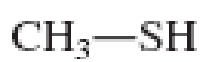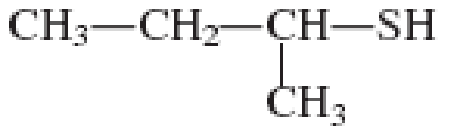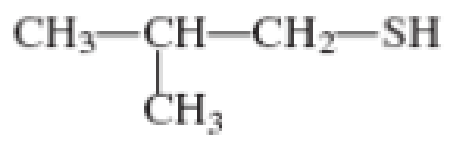Chapter 3, Problem 3.133EP### Organic And Biological Chemistry

7th Edition
STOKER + 1 other
ISBN: 9781305081079

#### Solutions

Chapter
Section### Organic And Biological Chemistry

7th Edition
STOKER + 1 other
ISBN: 9781305081079
Textbook Problem

# Assign a common name to each of the following thiols.(a)

Interpretation Introduction

Interpretation:

Common name for the thiol that is given has to be assigned.

Concept Introduction:

Common names are not IUPAC names.  For giving a common name for thiol, the term mercaptan is used as a suffix.  This is the older name for thiols.  The name of the alkyl group is given that is followed by the word mercaptan with a space between the two words.

Explanation

Given structure of compound is,

To obtain common name for the given thiol, the word mercaptan is added after the alkyl group with a space in between.  The alkyl group present in the given compound is methyl...

(b)

Interpretation Introduction

Interpretation:

Common name for the thiol that is given has to be assigned.

Concept Introduction:

Common names are not IUPAC names.  For giving a common name for thiol, the term mercaptan is used as a suffix.  This is the older name for thiols.  The name of the alkyl group is given that is followed by the word mercaptan with a space between the two words.

(c)

Interpretation Introduction

Interpretation:

Common name for the thiol that is given has to be assigned.

Concept Introduction:

Common names are not IUPAC names.  For giving a common name for thiol, the term mercaptan is used as a suffix.  This is the older name for thiols.  The name of the alkyl group is given that is followed by the word mercaptan with a space between the two words.

(d)

Interpretation Introduction

Interpretation:

Common name for the thiol that is given has to be assigned.

Concept Introduction:

Common names are not IUPAC names.  For giving a common name for thiol, the term mercaptan is used as a suffix.  This is the older name for thiols.  The name of the alkyl group is given that is followed by the word mercaptan with a space between the two words.

### Still sussing out bartleby?

Check out a sample textbook solution.

See a sample solution

#### The Solution to Your Study Problems

Bartleby provides explanations to thousands of textbook problems written by our experts, many with advanced degrees!

Get Started

#### Find more solutions based on key concepts